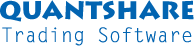#twitter-follow { border: 0;position: fixed; top: 240px; left:0;} #live-support { border: 0;position: fixed; top: 370px; left:0;} #knowledge-base { border: 0;position: fixed; top: 170px; left:0;}

### QuantShare Programming Language Tutorial

Updated on 2012-11-08

The QS programming language allows you to do different things in QuantShare. It can be used to:

- Plot candlesticks and indicators on a chart
- Create a composite indicator
- Scan stocks
- Create watchlists
- Implement fitness functions for the PBIL and genetic algorithm optimizers
- And more...

Introduction

A QuantShare formula is a collection of statements or commands, which tells QuantShare what to do and what to calculate.

Commands usually contain variables and functions:

Variables: The variables are at the core of any program. Their role is store information, so that the program can use that information later.
Not all variables are the same. Each variable can store a particular type of data. In QuantShare, we have two types of data: Numeric and Text.

Example:
- Create two variables: The first one stores the moving average data and the second one stores the close price.
- Compare the value of these variables. In other words, we are comparing the close price with the moving average.

Functions: A function is something you call in a program to perform a specific task. There are two different types of functions:
Function that performs a task and returns a value
Function that performs a task and do not return a value

Examples:
- The "SMA" function calculates the moving average of a stock and returns the moving average data.
- The "Plot" function plots an indicator on a chart. This function returns not values.

Different Elements of a QS formula

As we said above, a QuantShare formula is composed of a collection of statements.
Each statement must end with a semi-colon ";". This how the program recognizes that a statement ends.
Each statement is composed of several tokens. A token is the minimal lexical element of a programming language.

Here is a list of the different tokens:

Identifiers: Composed of variables and functions

Literals: Literals or constants are values that cannot be changed. The value of a literal is obvious because it is written in the program literally. There are two types of literals in QuantShare: Numeric (double) or Text (string).

Examples:
Numeric constant: 10
Text constant: "Hello"

Note that that "string" constants are always enclosed in quotes or single quotes. Otherwise, the program will think that this is a variable name or a function.

Operators: An operator is a symbol that represents a specific action.

The plus sign (+) is an operator that represents addition.
The basic mathematic operators are:
(-) => Subtraction
(*) => Multiplication
(/) => Division
-> Make these appears in a table

Relational and equality Operators:
< => Less than
> Greater than
<= Less than or equal to
>= Greater than or equal to
== Equal to
!= Not equal to

Assignment operator:
= Store the value returned by the expression after the "=" in the variable specified before "=".

Example:

a = 5 + 2;

Here is how it works:

The program finds the assignment operator (=) and then understands that it must store the value returned by the expression located at the right in the variable "a".
It then calculates that expression, which is a simple mathematical addition that returns (7).
It stores (7) in the variable (a).

Let us create another instruction:

b = a / 2;

When calculating the expression (a / 2). The program already knows that the variable "a" is equal to 7 and thus returns (3.5), which is equal to (7 / 2).
The value (3.5) is stored in the variable (b).

Punctuators: They are used to separate line of codes, variables etc. They are also called separators.

As we said previously, each statement must end with a semi-colon and therefore the semi-colon is a punctuator.

Other punctuators: ; , = ()

QuantShare Language Basics

As we said previously, identifiers are composed of variables and functions.

QuantShare has some built-in variables that identify specific price fields and which are: close, open, high, low, volume and openint.

Example using the assignment operator:

a = close;

// the variable "a" contains now the same data as the "close" built-in variable.

A QuantShare variable in fact does not store a single value (such as 18), it stores an array or a list of numeric/text values. This list contains the values that correspond to each trading bar.

Let us take the "close" variable as an example:

Here is how to the "close" variable looks like (Just an example):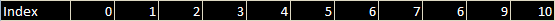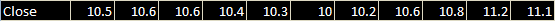Each index corresponds to a trading bar. If we are working with daily data, then each index correspond to a date.

Example: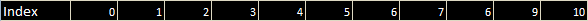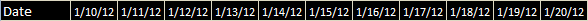For instance, the second index (1) corresponds to the date (1/11/2012) and the close price at that date is "10.6".

Using one-minute data, each index/bar correspond to a date/time.

Example: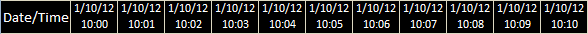Basic Operations

Operations, whether they are mathematical or relational are performed on each index.

If you add the value of two variables:

a = open + close;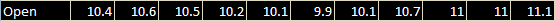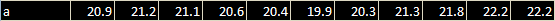Then for each index, the value of "open" and "close" will be added and a new array is associated to the "a" variable.

Another Example:

a = open > close;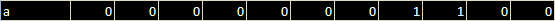"1" for true and "0" for false.

QuantShare Functions

A function is something you call in a program to perform a specific task.

For example, the "SMA" function calculates a simple moving average.

Function Syntax:
FunctionName([Parameters])

Each function has zero, one or more parameters. Parameters are variables or statements specified after the function name, inside the parentheses. They are passed to the function so that this function can use them to perform its calculation. Parameters are separated by colons ",".

For example, you can pass a period to the "SMA" function to specify the number of bars to use to calculate the moving average.
A 5-Bar simple moving average can be called as follows:

a = sma(5);

Here, we use the assignment operator to save the result of the "sma" function in the "a" variable.

The "a" variable now contains the moving average data.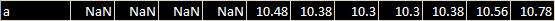Nan: Not a number
Because in the above example, the SMA function requires 5 elements to perform its calculation, it cannot calculate the moving average of the first four elements and this is why it sets the "NaN" value.

Popular Functions

Here are some of the most popular functions in QuantShare:

Ref:

References a previous or subsequent element in a ARRAY. A negative period references X periods in the future; a positive period references X periods ago

Example:

a = ref(close, 5);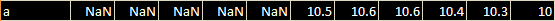Hhv:

For each trading bar, calculates the highest value over a specified period.

Example:
a = hhv(close, 5);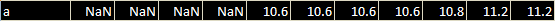Llv:

For each trading bar, calculates the lowest value over a specified period.

Example:
a = llv(close, 5);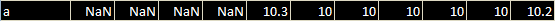Sum:

Calculates a cumulative sum of the ARRAY for the specified period
a = sum(close, 5);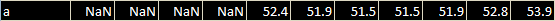Note that there are more than 200 built-in functions in QuantShare. In the formula editor, type a variable name, the assignment operator (a = ) then click on CONTROL+SPACE to display the list of available function.

After you type the indicator name and the first parentheses (Example: "a = sma(" ), you will see a tooltip above the function name. This tooltip shows a brief description of that function and it also shows you the parameters that are required by that function.

Besides, you can sometimes see something like "1 of 2" or "1 of 3" before the function name. This means that the function can accept several combinations of parameters. Click on the tooltip to display the other parameter combinations.

Example:

Type: a = sma(
In the tooltip, you can see:

1 of 2 Number Sma(~Number timeperiod)

This means that the "Sma" function has two different parameter combinations.
The first "Number" means that it returns a numeric variable (array) and that it accepts only one parameter (numeric) that corresponds to the time period.

After you click on that tooltip, you will see:

2 of 2 Number Sma(~Number close, ~Number timeperiod)

This is the second definition of the "Sma" function. It accepts two parameters (close and timeperiod) and returns a numeric variable.
In the second definition, you can specify the time-series that will be used to calculate the moving average. In the first definition, the "close" series is used by default. In the second one, you can specify a custom time-series.

Definition 1: a = sma(5);
Definition 2: a = sma(close, 5);

The above instructions return the same data.

Here is another example based on the second definition:
a = sma(sma(10), 5);

The above instruction calculates the 5-bar moving average of the 10-bar moving average.

It is the same as the following formula:
a = sma(10);
a = sma(a, 5);

Instead of passing the 10-bar sma function directly in the first parameter, we store the data in the variable "a" and pass it to the second "sma" function.

Difference between Assignment and Equality

The assignment operator is something very important. It lets us assign the result of the expression on the right side of the operator to the variable on the left side.

In the example below, the value of the moving average is assigned to variable "a" and the variable "a" is assigned to variable "b".

a = sma(10);
b = a;

At the end, the value of "b" is equal to "a" and it is equal to SMA (simple moving average).

The equality operator (==) is used to check whether the two expressions on both sides are equal or not. It compares each element of the array individually and returns true (or
"1") of they are equal, and false (or "0") if they are not.

Example:

a = close == 10;

The above function uses both the assignment and the equality operators.
It first compares the close array with the value (10) then assigns the result of that expression to the variable (a)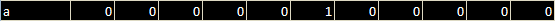Search PostsRecent Posts Chart Layouts Explained - With Custom Scripts Posted 735 days ago Create Graphs using the Grid Tool Posted 915 days ago Profile Graphs Posted 1020 days ago More about QuantShare Programming Language Posted 1099 days ago Backtest Trading Strategies Programmatically and on Schedule Posted 1245 days ago QuantShare Trading Software: New Features in the 3.7.0 Version Posted 1275 days ago Compare Multiple Securities in a Single Chart Posted 1335 days agoPrevious Posts QuantShare Programming Language Tutorial Posted 3862 days ago Sentiment Analysis: How to measure the sentiment score of your stock tweets Posted 3869 days ago Running QuantShare on Amazon's EC2 Cloud & Automate Strategies Signals Posted 3875 days ago Money Management: Optimize the scale-in strategy Posted 3878 days ago Money Management: Scale-in Trading Strategy Posted 3891 days ago How to measure market strength with the composite tool Posted 3898 days ago Download historical EOD data for the stock, futures, ETF and Forex markets Posted 3912 days ago How to build and backtest a robust stock trading system Posted 3921 days ago Backtesting chart patterns using the auto support and resistance indicator Posted 3926 days ago German Stock Exchanges: 5 free ways to download historical EOD/Intraday data Posted 3932 days ago How to use QuantShare screener to trade penny stocks Posted 3935 days ago Long Term Trading with Technical Analysis Posted 3941 days ago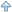Back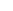Support Contact Us Trading Forum How-to Lessons Manual Company About Us Privacy Terms of Use Copyright © 2023 QuantShare.com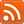Trading financial instruments, including foreign exchange on margin, carries a high level of risk and is not suitable for all investors. The high degree of leverage can work against you as well as for you. Before deciding to invest in financial instruments or foreign exchange you should carefully consider your investment objectives, level of experience, and risk appetite. The possibility exists that you could sustain a loss of some or all of your initial investment and therefore you should not invest money that you cannot afford to lose. You should be aware of all the risks associated with trading and seek advice from an independent financial advisor if you have any doubts.